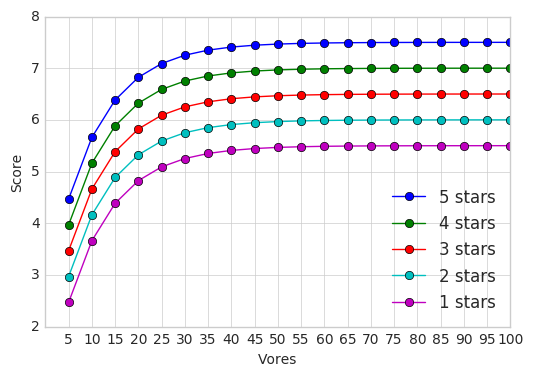# Weighted Rating¶

I was looking for an algorithm that takes into account the number of votes and not just the average rating. For example a 4 star review with 100 reviewers should account for more that 5 star review from 3 people.

A bit of googling found an answer on mathexchange. The formula given there is:

$score = 5p/10 + 5(1 -e^{-q/Q})$

Where

• p is the rating
• q is the number of votes
• Q is the weight of number of votes

This looked good but I wanted to have a quick look on how this behaves.

In :
import numpy as np
import matplotlib.pyplot as plt

%matplotlib inline
plt.style.use('seaborn-whitegrid')

In :
def weighted_score(rating, votes, weight=10):
return (5 * rating/10.0) + (5 * (1 - np.e**(-votes/weight)))

In :
for rating, votes in [(5, 10), (4, 1000)]:
print('rating of %d from %d people = %.2f' % (rating, votes, s))

rating of 5 from 10 people = 5.66
rating of 4 from 1000 people = 7.00

In :
ratings = [5, 4, 3, 2, 1]
for rating in ratings:
scores = [weighted_score(rating, vote) for vote in votes]
plt.plot(votes, scores, '-o', label='%d stars' % rating)